Java ProgrammingWhat are expression, blocks, conditional and branching statements in java

# What are expression, blocks, conditional and branching statements in java

In this tutorial we will learn about expression, blocks, conditional and branching statements along with their implementation in java.

• Detailed explanation about what are expression,blocks, conditional and branching statements in java is given below :

1. Expressions:- For building the blocks of any java program, expressions are used. It is built using operators, variables, values and method calls. In java, regular expression defines the pattern of a string. It can be used to edit, manipulate and search text. It differ’s slightly for each language but it is not language specific.

1. Statement: – It is nothing but more than one expression followed by semicolon. The group of statement is called as statement block. It may also include variable declarations.

1. Control Statements: –

1. If Statement: – Conditionally execute single statement.

1. If-Else Statement: – Conditionally select one of the two statements and execute it.

1. Switch Statement: – Conditionally choose one of the several statements and execute it.

1. Repetitive Statements: – It has three types of loop statements: for loop, while loop, do while loop. These statements are allowed as nested statement to be executed repetitively. Each execution of nested statement is called iteration of the loop. These iterations are controlled by a boolean expression. In while loops and for loops boolean expression is evaluated before each iteration. In do-while loop boolean expression is evaluated after each iteration. If the boolean expression becomes false the loop is terminated.

1. While Loop: – In this case boolean expression, can be true or false. It is a pretest loop statement. The boolean expression is tested start of the each iteration of the loop. The loop terminates when it is false.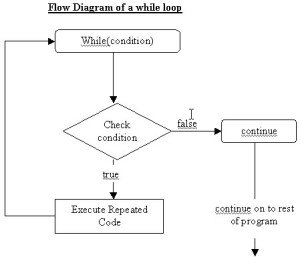Example : This example shows how while loop is used in a class.

```public class While {//create class
public static void main(String args[]){//main method
int num = 16; //define integer num
while (num < 20) {
{
/*check condition num is greater than define integer or not if true then print num*/
System.out.println ("Value of num:" + num); //print statement
num ++;
}
}
}//num increment by one
```

Output :

```Value of num: 16
Value of num: 17
Value of num: 18
Value of num: 19
```

1. For Loop: – It is a pretest loop statement. In this case initialization and increment is an expression. A boolean expression also is an expression that can be true or false. In this case when loop is executed then first initialization expression is evaluated. This expression assigns the initial value to loop control variable (eg. j=1).
The boolean expression is tested start of the each iteration of the loop. The loop terminates when it is false and expression is frequently a comparison (j<=10).
After that increment expression is evaluated, that can increment the control variable (eg. j++).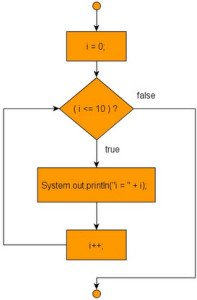Example : This example shows how to use for loop in a class.

```public class ForLoop {
public static void main(String[] args) {
// Initialization is executed only once.
//loop is executed till condition is true
for(int num = 0; num <= 2 ; num++)
//print the num value
System.out.println ("Number is: " + num);
}
}
```

Output :

```Number is: 0
Number is: 1
Number is: 2
```

1. Do-While Loop:- It is a post-test loop statement. In this case boolean expression is tested after each iteration of the loop. If it is false that means loop is terminated.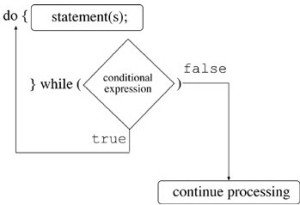Example : This Java Example shows how to use do- while loop to iterate in Java program.

```public class DoWhile {
public static void main(String[] args) {
//In this case the condition is checked after executing the loop body.
//So loop will be executed at least once even if the condition is false.
int a =0;  //declare int value in variable i
do{//start do loop
System.out.println ("a is: " + a); //print a
a++;//increment a by 1
//if the condition is false then execute while loop
}while(a < 4); }}
```

Output :

```a is: 0
a is: 1
a is: 2
a is: 3
```

1. Special Control Statements: –

1. Return Statement: – It is used to terminate execution of the method and in the definition of a method to initialize return value.
It can be used in two ways:

• Method with returned type void.

• Method with returned type non-void.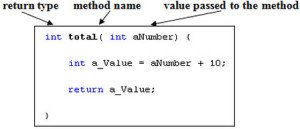Example : This example shows how to write return in a class.

```public class Return
{
//declare integer with static

static int a=5;
static int b=11;
public static void main(String[] args)//void is used
{
System.out.println (a+b); //addition of a and b
return;//return give addition of a and b
}
}
```

Output :

`16`

1. Continue Statement: – This statement is used to terminate an iteration of the loop in for loop, while and do-while loop.
The boolean expression and its increment are evaluated after the continue statement is executed in a for loop. In this case nested statements are executed again if and only if the boolean expression is true.
The boolean expression is evaluated after the continue statement is executed in a while loop and do-while loop. In this case nested statements are executed again if and only if the boolean expression is true.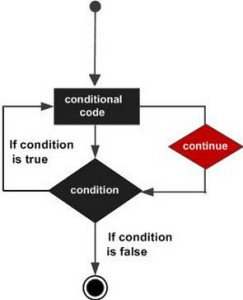Example : This example shows how to use java continue statement to skip the iteration of the loop in a class

```public class Continue
{
public static void main(String args[]) {
//use array to declare integer
int [] num = {1,14,9,16,5,7};
for(int a : num ) {//store num values in int a
//check if a equals to 16 then skip 16 and print next values
if( a == 16 ) {
continue ;
}//skip 16 and continue iteration
System.out.println (a); //print statement
System.out.println ("\n");
}
}
}
```

Output :

```1
14
9
5
7
```

1. Break Statement : – It is used to terminate execution of the statement in loop statements like for loop, while loop, do-while loop and also in switch statements.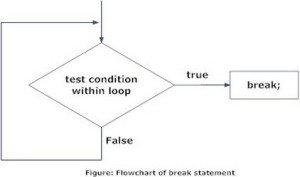Example : This Java Example shows how to break the loop or condition in a class.

```public class Break{
public static void main(String[] args)
{
int j,k;
System.out.println ("Addition between 1 to 8 :");
/*check j is less than 8 then it increment
with 1 and go to next loop and check k is
less than j then go to if loop addition of
j and k equal to 6*/
for (j  = 1; j < 8; j++)
{
for (k = 2; k <j; k++)
{
if (j+k==6) {
break ;
}//break if condition is false
}
if (j==k)
{
//check j equal to k if yes then print j
System.out.println (" "+j);
}
}
}
}
```

Output :

```Addition between 1 10 8:
2
3
5
6
7
```

1. Blocks :- A block is nothing but the group of zero or more than zero statements between balanced braces.

Example :

```public class Block {
static int a=10;
static int b=20;
public static void main(String[] args) {
boolean condition = true;
if (a==b)
{ 	// begin block 1
System.out.println ("Condition is true.");
} 	// end block 1
else
{	 // begin block 2
System.out.println ("Condition is false.");
}	 // end block 2
}
}
```

Output :

`Condition is false.`

1. Loops and Branching : –

• Loops: –
1. do : – In this case, do loop body is always executed at least once. Semicolon is always required after the conditional expression.

Example : This Java Example shows how to use do while loop to iterate in Java program.

```public class DoWhile
{
public static void main(String[] args) {
//In this case the condition is checked after executing the loop body.
//So loop will be executed at least once even if the condition is false.
int a =0;  //declare int value in variable i
do
{//start do loop
System.out.println ("a is: " + a); //print a
a++;//increment a by 1
//if the condition is false then execute while loop
} while (a < 4) ;
}
}
```

Output :

```a is: 0
a is: 1
a is: 2
a is: 3
```

1. while : – This keyword indicates that the loop is executed continuously upto condition is true.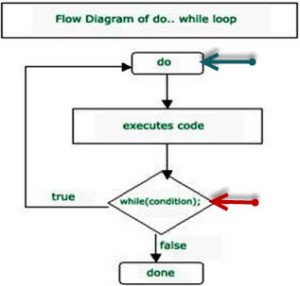Example :

```public class While {//create class
public static void main(String args[]){//main method
int num = 16; //declare integer num
while (num < 20) {{
/*check condition num is
greater than define integer or not if
true then print num*/
System.out.println ("Value of num:" + num); //print statement
num ++;
}
}
}//num increment by one
```

Output :

```Value of num: 16
Value of num: 17
Value of num: 18
Value of num: 19
```

1. for : – It is a pretest loop statement. In this case initialization and increment is an expression. A boolean expression also is an expression that can be true or false. In this case when loop is executed then first initialization expression is evaluated. This expression assigns the initial value to loop control variable (eg. j=1). The boolean expression is tested start of the each iteration of the loop. The loop terminates when it is false and expression is frequently a comparison (j<=10). After that increment expression is evaluated, that can increment the control variable (eg. j++).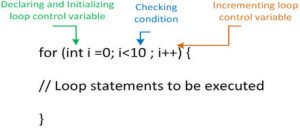Example : This example shows how to use for loop in a class.

```public class ForLoop {
public static void main(String[] args)
{
// Initialization is executed only once.
//loop is executed till condition is true
for(int num = 0; num <= 2 ; num++)
//print the num value
System.out.println ("Number is: " + num);
}
}
```

Output :

```Number is: 0
Number is: 1
Number is: 2
```

• Branching : –

1. break : – The break keyword is used to exit a for loop, while loop, do loop or to the end of a case block in a switch statement.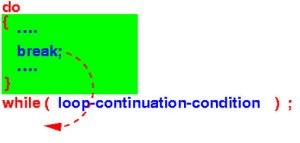Example : This Java Example shows how to break the loop or condition in a class.

```public class Break{
public static void main(String[] args){
int j,k;
System.out.println ("Addition between 1 to 8 :");
/*check j is less than 8 then it increment
with 1 and go to next loop and check k is
less than j then go to if loop addition of
j and k equal to 6*/
for (j  = 1; j < 8; j++) {
for (k = 2; k <j; k++) {
if (j+k==6)
{
break ;
}//break if condition is false
}
if (j==k)
{
//check j equal to k if yes then print j
System.out.println (" "+j);
}
}
}
}
```

Output :

```Addition between 1 10 8:
2
3
5
6
7
```

1. continue : – Continue is helpful for skipping the next iteration of a do, while or for loop statement.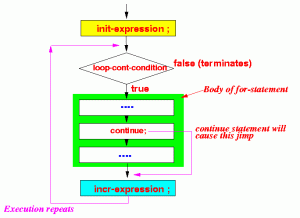Example : This example shows how to use java continue statement to skip the iteration of the loop in a class

```public class Continue
{
public static void main(String args[]) {
//use array to declare integer
int [] num = {1,14,9,16,5,7};
for(int a : num ) {//store num values in int a
//check if a equals to 16 then skip 16 and print next values
if( a == 16 ) {
continue ;
}//skip 16 and continue iteration
System.out.println (a); //print statement
System.out.println ("\n");
}
}
}
```

Output :

```1
14
9
5
7
```

1. Hence, we now have sound knowledge about what are expression, blocks, conditional and branching statements in java

### Top 10 cyber security threats that arose last year

21,501Fans
4,218Followers
103,000Subscribers

### Top 10 cyber security threats that arose last year

- Advertisement -